# Math 222 MATLAB Supplementary lecture

## Part 3: Euler's method

Section 2.7 of Boyce and DiPrima covers the Euler method for approximating a solution to the differential equationGiven an initial condition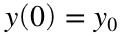, the method gives a sequence of approximations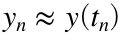defined by a recurrence relation.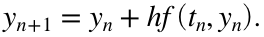where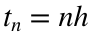and h is the time step chosen by the user.
Now we're ready for the real thing. Let's solve the problemThis has exact solution (which is easy to find because the ODE is separable)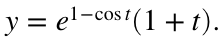tFinal = 2*pi;
N = 100;
h=tFinal/N;
t=linspace(0,tFinal,N+1); % type 'help linspace' to see how this works
y=zeros(1,N+1);
I want to point out the syntax used to define yExact in the above line. In particular the .* notation which is used for elementwise multiplication, since exp(1-cos(t)) and (1+t) are each vectors. You will need this to form the exact solution below, you will also need to use the .^ notation to compute an elementwise power.
y(1) = 1; % setting the initial condition
for n=1:N
y(n+1) = y(n) + h * f(t(n),y(n));
end
yExact=exactFunction(t);
plot(t,y,t,yExact,'--'); xlabel('t'); ylabel('y'); title('Look, ma! I solved an ODE in MATLAB!');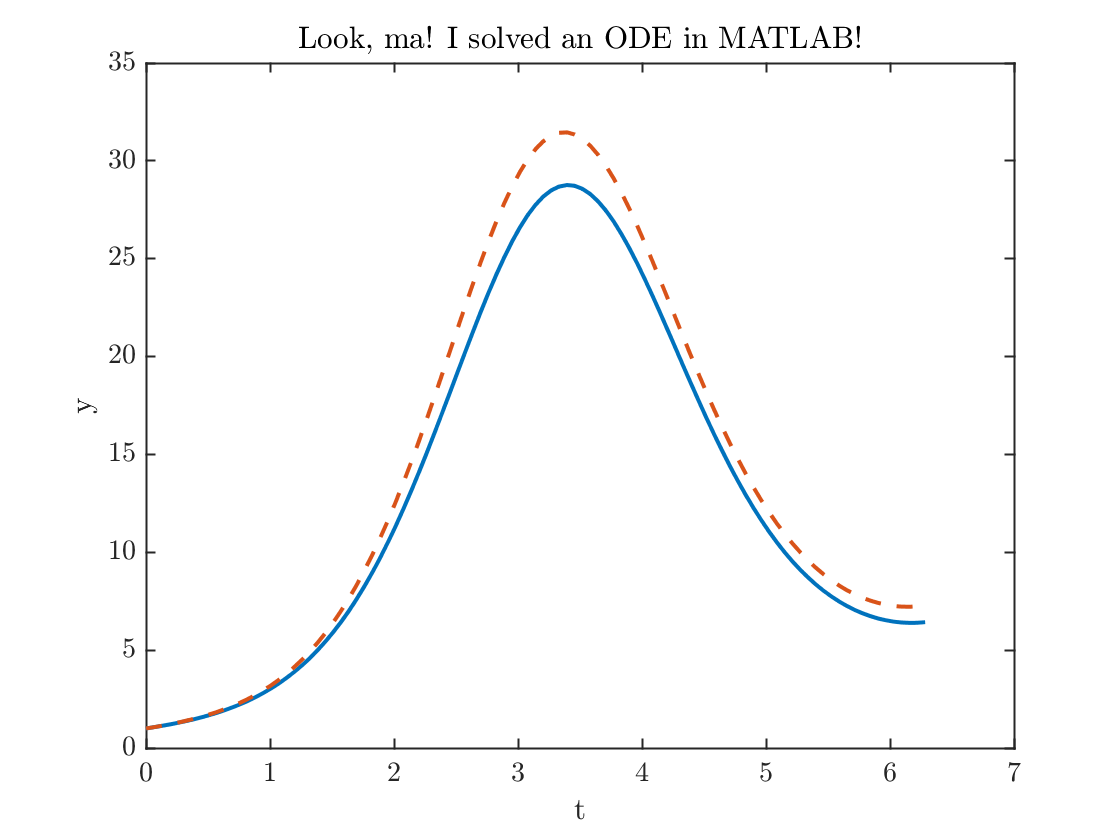error100= max(abs(y-yExact));
fprintf('The maximum error = %0.2f.\n',error100)
The maximum error = 2.85.
Some observations:
• I used the max function to evaluate the error.
• The code looks very neat because I use the call to the function f(t(n),y(n)) defined at the bottom of this file, instead of writing out the whole formula inside my loop.
• I used the fprintf command to format the output. Get help on this function by typing help fprintf at the MATLAB >> prompt.
That error is a little bit larger than I'd like, let's double the number of points and try again
N = 200;
h=tFinal/N;
t=linspace(0,tFinal,N+1); % type 'help linspace' to see how this works
y=zeros(1,N+1);
y(1) = 1; % setting the initial condition
for n=1:N
y(n+1) = y(n) + h * f(t(n),y(n));
end
yExact=exactFunction(t);
plot(t,y,t,yExact,'--')
xlabel('t'); ylabel('y'); title('Look, ma! I solved it even better!');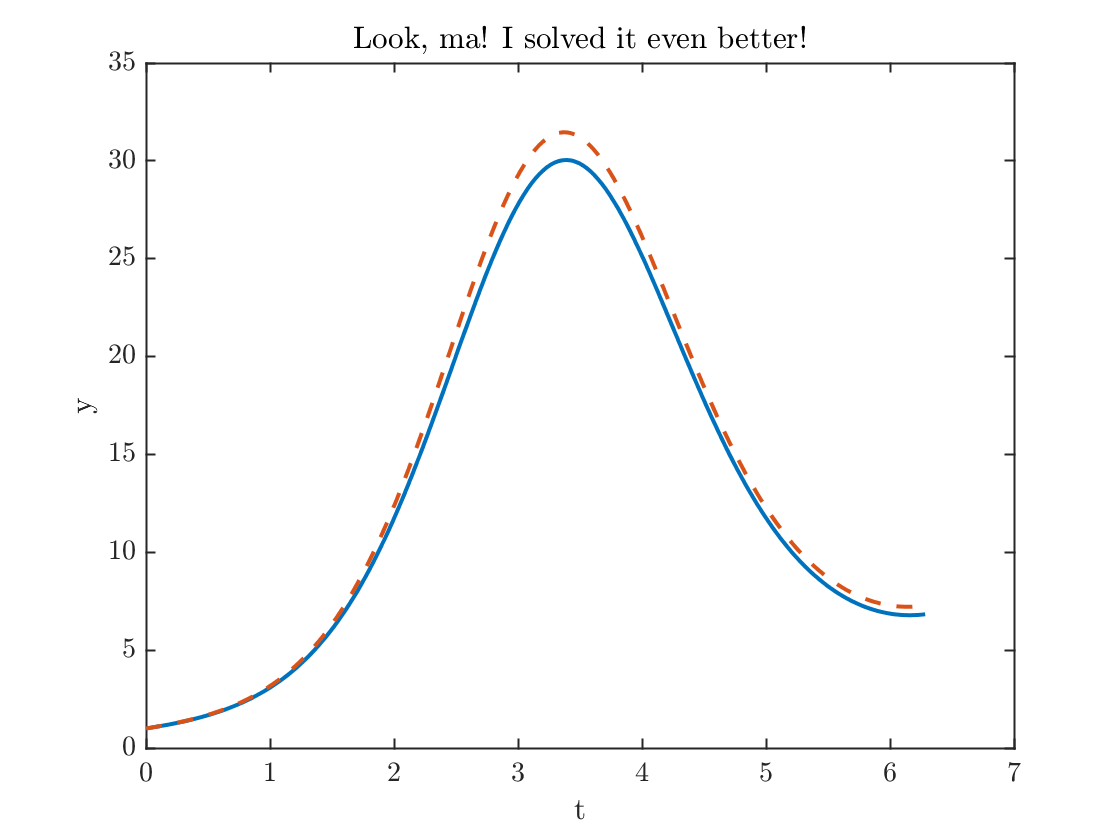error200= max(abs(y-yExact));
fprintf('The maximum error = %0.2f.\n',error200)
The maximum error = 1.49.

#### Demonstration of first order accuracy

The Euler method is a first-order method. This means that the error is proportional to the step size, that is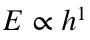. (The word first-order refers to the one in the exponent). This means that if we divide h by 2, then the error should be (approximately) cut in half. We have just done that, so let's compare the errors:
fprintf('The maximum error went down by a factor of %f.\n',error100/error200);
The maximum error went down by a factor of 1.907074.
That's pretty close!. As h is taken smaller and smaller, this ratio will get closer to 2.
Again, note how useful it is that I call the function f(t,y) in my loop instead of writing out the formula. This is good programming style, because if I decide to change the definition of f, I only have to change it one time, instead of multiple times.

### Functions used

Right hand side of ODE
function yprime=f(t,y)
yprime = (sin(t)+1/(1+t))*y;
end
Exact solution to the ODE
function x = exactFunction(t)
x= exp(1-cos(t)).*(1+t);
end